Register
 Email: Passwd 验 证:换一张 Have you forgotten your password? Stay signed in Log In
 ALL Title Author Keyword Sponsors Type Publication date Submitted Time Subject Conference Name Source Categories KOS Subject Advisor ORCID Advanced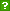ISCAS OpenIR  > 软件所图书馆  > 期刊论文
 Title: On the Gap Between Trivial and Nontrivial Initial Segment Prefix-Free Complexity Author: Baartse, Martijn ; Barmpalias, George Keyword: Kolmogorov complexity ; Initial segment prefix-free complexity ; K-triviality ; Low for Omega Source: THEORY OF COMPUTING SYSTEMS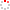Issued Date: 2013 Volume: 52, Issue:1, Pages:28-47 Indexed Type: SCI Department: [Baartse, Martijn] Tech Univ Cottbus, Inst Comp Sci, D-03046 Cottbus, Germany. [Barmpalias, George] Chinese Acad Sci, State Key Lab Comp Sci, Inst Software, Beijing 100190, Peoples R China. Abstract: An infinite sequence X is said to have trivial (prefix-free) initial segment complexity if the prefix-free Kolmogorov complexity of each initial segment of X is the same as the complexity of the sequence of 0s of the same length, up to a constant. We study the gap between the minimum complexity K(0(n)) and the initial segment complexity of a nontrivial sequence, and in particular the nondecreasing unbounded functions f such that K(X (sic)(n)) <= K (0(n)) + f (n) + c for a constant c and all n (*) for a nontrivial sequence X, where K denotes the prefix-free complexity. Our first result is that there exists a Delta(0)(3) unbounded nondecreasing function f which does not have this property. It is known that such functions cannot be Delta(0)(2) hence this is an optimal bound on their arithmetical complexity. Moreover it improves the bound Delta(0)(4) that was known from Csima and Montalban (Proc. Amer. Math. Soc. 134(5): 1499-1502, 2006). Our second result is that if f is Delta(0)(2) then there exists a non-empty Pi(0)(1) class of reals X with nontrivial prefix-free complexity which satisfy (*). This implies that in this case there uncountably many nontrivial reals X satisfying (*) in various well known classes from computability theory and algorithmic randomness; for example low for Omega, non-low for Omega and computably dominated reals. A special case of this result was independently obtained by Bienvenu, Merkle and Nies (STACS, pp. 452-463, 2011). English Abstract: An infinite sequence X is said to have trivial (prefix-free) initial segment complexity if the prefix-free Kolmogorov complexity of each initial segment of X is the same as the complexity of the sequence of 0s of the same length, up to a constant. We study the gap between the minimum complexity K(0(n)) and the initial segment complexity of a nontrivial sequence, and in particular the nondecreasing unbounded functions f such that K(X (sic)(n)) <= K (0(n)) + f (n) + c for a constant c and all n (*) for a nontrivial sequence X, where K denotes the prefix-free complexity. Our first result is that there exists a Delta(0)(3) unbounded nondecreasing function f which does not have this property. It is known that such functions cannot be Delta(0)(2) hence this is an optimal bound on their arithmetical complexity. Moreover it improves the bound Delta(0)(4) that was known from Csima and Montalban (Proc. Amer. Math. Soc. 134(5): 1499-1502, 2006). Our second result is that if f is Delta(0)(2) then there exists a non-empty Pi(0)(1) class of reals X with nontrivial prefix-free complexity which satisfy (*). This implies that in this case there uncountably many nontrivial reals X satisfying (*) in various well known classes from computability theory and algorithmic randomness; for example low for Omega, non-low for Omega and computably dominated reals. A special case of this result was independently obtained by Bienvenu, Merkle and Nies (STACS, pp. 452-463, 2011). Language: 英语 WOS ID: WOS:000316087100003 Citation statistics: Content Type: 期刊论文 URI: http://ir.iscas.ac.cn/handle/311060/16700 Appears in Collections: 软件所图书馆_期刊论文

 Files in This Item:

There are no files associated with this item.

 Recommended Citation: Baartse, Martijn,Barmpalias, George. On the Gap Between Trivial and Nontrivial Initial Segment Prefix-Free Complexity[J]. THEORY OF COMPUTING SYSTEMS,2013-01-01,52(1):28-47.
 Service Recommend this item Sava as my favorate item Show this item's statistics Export Endnote File Google Scholar Similar articles in Google Scholar [Baartse, Martijn]'s Articles [Barmpalias, George]'s Articles CSDL cross search Similar articles in CSDL Cross Search [Baartse, Martijn]‘s Articles [Barmpalias, George]‘s Articles Related Copyright Policies Null Social Bookmarking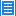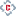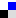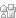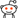您对该条目有什么异议，请填写以下表单，管理员会尽快联系您。 `内 容：` Email： * 单位： 验证码：刷新
 您在IR的使用过程中有什么好的想法或者建议可以反馈给我们。 `标 题：` * `内 容：` Email： * 验证码：刷新Copyright © 2007-2022  中国科学院软件研究所 - Feedback Powered by CSpace